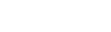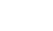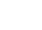# Decision Trees & Random Forests: Deep DiveWritten by:
Dennis Turp (Data Scientist at Modulos)

If you’ve always wondered how decision trees and random forests work, as well as what problems they can solve, this blog is here to answer your questions! We will illustrate these concepts by using the practical example of a real estate company wanting to predict housing prices.

#### A practical example: Predicting housing prices

Let’s imagine that we are an employee of a real estate company, and we are tasked with developing a method to determine property prices (data source — external link). Features used to determine the property price could include the year the house was built and / or the size of the property. Let’s also assume that we have data on historical property sales and call this our dataset X.

Table 1 shows a small part of our dataset. In addition to the mentioned features – year of construction, size of property and price – further information in this dataset include the type of foundation and heating system, the number of bathrooms, etc. The entire dataset contains over 80 features (columns) and over 1400 historic housing sales (rows).Table 1: Example data of historic housing sales. The “SalePrice” column is the price of the house e.g. the quantity we want to predict. All other columns contain metadata.
###### Developing a predictor for the property value

How would we go about developing a reliable method to predict the price of a property based on the given dataset?

One of the first thoughts that probably comes to mind is splitting our big dataset of historic housing sales into smaller groups which will contain similar houses. In order to do that, we need to specify what we mean by similar. We could split our historical dataset X based on the year of construction. For example, houses built before 1980 would go to one group, while those built after 1980 would go to the other group. If we split our dataset according to this rule and look at the distribution of housing prices in the two groups, we can see that older houses tend to be cheaper than newer houses (see Figure 1).Figure 1: Graph showing the distribution of housing values for the split data. The blue and orange distributions show the price of houses built before and after 1980, respectively. Blue houses built before 1980 and orange houses built after 1980. We can see that newer houses tend to be more expensive. This indicates that the year of construction might be an important factor in determining the housing price.
###### Further refining the predictor

This intuitively seems to be correct and we can conclude that the year of construction is a rough indicator of the price. To get a more accurate estimate, we can consider other factors that might be key in determining the price. We could split our two groups further by looking at the size of the property, then split them based on the heating system, etc. If we keep splitting our dataset and sub-datasets based on the rules that we come up with, we will end up with several chunks of the dataset, each containing houses with similar features.

By splitting our dataset based on those rules, we’ve essentially built our first decision tree (see Figure 2; sequence of splits).Figure 2: Graph showing how we build a decision tree by splitting the data into different groups.

To infer the price of a new house, we would simply use the rules that we deduced and follow the respective branches of the decision tree until we arrived at one of the leaves. For instance, if we wanted to determine the price of a house which was built before 1980 on a property smaller than 10,000 units, we would end up in chunk 3. The idea is that the average price of the resulting leaf subset will be a good estimate of the actual price of the new house.

#### Building decision trees

But how do we know if the rules that we’ve defined are optimal? Why wouldn’t we split the dataset by houses that were built before 1960 instead of 1980? Furthermore, how many splits should we perform?

These are some of the questions that decision tree learning algorithms aim to answer. One of the popular ways to grow a decision tree is using the CART algorithm. It determines how to split the data into subsets by using statistical information of previously sold houses.

###### CART algorithm

For every node (branch point) in the tree:

1. Find the best split for each column/feature:
• Sort the column/feature entries from smallest to largest
• Perform all possible splits (if the training dataset contains K different values we have (K-1) possible splits)*: for categorical values we have 2^(K-1)-1 possible splits.
• Measure the quality of each split by using a metric suited for your problem (see discussion on metrics below)
• Select the best split for this column / feature by comparing the scores computed in the previous step
2. Select the column/feature with the best split.
3. Repeat steps 1) and 2) until you hit a stopping criteria. Possible stopping criteria could be the minimum number of samples/data points in a leaf (last node) or the maximum depth (the number of splits performed after each other)

A question that remains is how to measure the quality of a split.

The answer depends on the task. For classification tasks, common metrics are “Gini impurity” and “Entropy”. For a regression task such as ours, common metrics are the “Mean squared” error and the “Median absolute” error.

###### Regression
• Mean squared error (Variance reduction)
The mean squared error is equivalent to computing the variance of all samples that end up in one leave, hence its other name – variance reduction. Generally, the lower the score, the better.
The equation above describes the mean squared error, where:
•are all the samples that end up in leave m,
•is the total number of samples that end up in leave m,
•is the average of all the samples that ended up in one leave.
• Median absolute error
Similar to the mean squared error, but with the median instead.

#### What are the problems with decision trees and how can random forests help out

One of the major problems with fitting decision trees is that they are likely to overfit to the historical data. What do we mean by that?

Imagine again that you are looking at data of historical housing sales. While houses were mostly sold at reasonable prices, an occasional buyer might have misjudged the price and paid much more than the average. The real estate company does not want to repeat this mistake. As our tree grows in depth, the rules become more and more specific, and eventually a rule that splits off this expensive house into a leaf will be found. Deep trees are more likely to overfit to a finite training set, because the leaves only contain a few samples and the partition of the dataset is very fine-grained.

The metric would also be minimal if all samples from the historic dataset were placed in their own leaf. To prevent decision trees from overfitting, one can, for example, set a minimum number of samples required at a leaf node or setting the maximum depth of the tree. Another way which proved to enhance stability is building ensembles of different decision trees, also known as a forest.

###### Sources of randomness

Since the CART algorithm is deterministic, one usually uses two sources of randomness to build a diverse set of decision trees that help reduce the variance of the predictions (Random Forests).

The first source of randomness comes from perturbing the data used at the root node. This is done by sampling new datasets where samples are drawn with replacement from the original dataset (also called bagging). This means the same data points can appear several times in the newly formed datasets.

The second source of randomness comes from the amount of columns / features considered when building the decision tree.

We build a new decision tree for every dataset that we construct with these two sources of randomness. Individually, these decision trees give suboptimal results. When we average those results to build a forest, we can observe that in a lot of cases, errors cancel each other out. We are less likely to overfit to single samples in the dataset.

###### Random Forest hyperparameters

Different random forests can still give different results, not only because of the randomness, but also because of different hyperparameters that we have to set. Key parameters that need to be adapted in order to find and train an optimal random forest for a new dataset are:

• The number of trees in the forest
• The splitting metric to use when building individual decision trees
• The number of random features to use when building new datasets
• The number of depths we want individual trees to grow to

#### Random Forests in Modulos AutoML

The question that arises now is how to choose the optimal hyperparameters; do we need to preprocess our data to use a random forest algorithm? Is our problem even solvable with a random forest algorithm? How do we adapt to changes that appear in our data over time?

These are all valid questions which can have a tremendous impact on the performance of the model and reaching the solution for the problem at hand. With the Modulos AutoML platform, we do our best to resolve these questions in a systematic way. If you would like to know more, get in touch with us.

If you found our explanation of decision trees and random forests insightful, feel free to share it!